### الصفحات

منصة تحفيظ القران الكريم اون لاين

https://quranmo.com

# بنك اسئلة مقدمة في الحاسب

file_1655744318036CSC- 101 INTRODUCTION

TO COMPUTERS

Question Bank Set - 1

MULTIPLE CHOICE ON VARIABLES 2

CODING EXERCISE ON VARIABLES 13

MULTIPLE CHOICE ON OPERATORS 14

MULTIPLE CHOICE ON DATA STRUCTURES 24

MULTIPLE CHOICE ON CONTROL FLOW 38

MULTIPLE CHOICE ON TYPECASTING AND ESCAPE SEQUENCES 41

MULTIPLE CHOICE ON VARIABLES

1. # What is wrong with the following code?

number_of items = 10

1. Nothing is Wrong

2. Variable Name is Wrong

3. I don’t know

4. Underscore is not allowed

2. # What type of variable it is?

x = "2.48"

1. Integer

2. Float

3. String

4. Double Float

3. # What type of variable it is?

wrong = False

1. Float

2. Bool

3. Integer

4. String

4. # What is the output of the following program?

a) \$x = 100

1. print(\$x)

A. 100

B. \$100

C. 100.0

D. Syntax Error

5. # What is the output of the following program? a) x1 = 100

b) x2y = 200

1. print(x1)

2. print(x2y)

A. 200

100

B. 100

200

C. 100 200

D. Syntax Error

6. Which one of these is a floating point number?

A. 3.2

B. 4

C. 500

D. “500.45”

7. Which of these will output the result 36? A: 30+*6

B: 6^6

C: 6**6 D: 6*6

Ans: D

8. In Python 3, what is the output of 1/2 ?

1. 1

2. 0

C. 0.5

D. None of the Above

Ans: C

9. What is printed by? print(3**2+1)

1. 7

2. 28

3. 10

4. 16

5. None of the above.

10. What is printed by?

val1 = 2

val2 = 3

val1, val2 = val2, val1 print(val1, val2)

1. 3 2

2. 2 3

3. 2

4. 3

5. None of the above.

11. .What is printed by this sequence of instructions?

x = 0

y = 1

z = 1

print(x or (y and not z))

1. 1

2. 0

3. True

4. False

5. None of the above.

1. What is printed by? a = 30

if a == 3 or not(a < 20): print("Yes")

else:

print("No")

1. Yes

2. No

3. a is 3

4. None

5. None of the above.

2. # What is the correct way to add a comment in Python?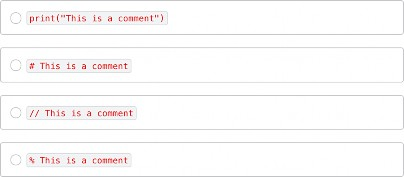3. # What is the correct way to set a variable 's' equal to the string "Hello World"?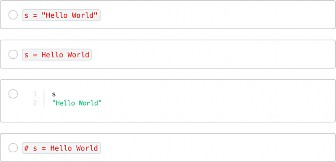4. # What type of variable is this?

a = True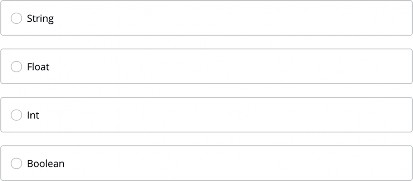5. # What type of variable is this? x = 100.45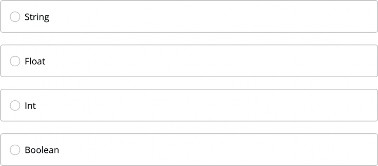6. What is printed by: print(2**2*2)

1. 15

2. 8

3. 16

4. 6

5. None of the above

7. Given

value = input("Input a number: ")

What is the type of value if 7 is input at the prompt?

1. int

2. str

3. float

4. long

5. None of the above

8. What is printed by? print(3**2+1)

1. 7

2. 28

3. 10

4. 16

5. None of the above.

9. What is printed by?

val1 = 2

val2 = 3

val1, val2 = val2, val1

print(val1, val2)

1. 3 2

2. 2 3

3. 2

4. 3

5. None of the above.

10. What is printed by: print(5**2)

1. 10

2. 25

3. 5

4. True

5. None of the above

11. Fill in the Blank to Print “Hi”

>>>>     (“Hi”)

1. Printf

2. Println

3. Output

4. Print

12. Which of the these will not be stored as a float a) 7.0

b) 7

c) 7/2

d) All the Above

13. Fill in the Blank with the output of this code

>> 1 + 2 + 3 + 4.0 + 5

>>

a) 15.00

b) 15

c) 15.0

d) None of the Above

14. Fill in the Blank to make this code correct

>> (1+ ) ** 2 16

a) 3

b) 3.0

c) 2

d) 2.0

15. What is the Output of this code

>> print(‘print(“print”)’)

1. An Error Message

2. ‘print(“print”)

3. print(“print”)

4. “print(‘print’)”

16. Fill in the blank to prompt for user input

>>>      (“Enter a number”)

1. Print

2. Scan

3. Input

4. Output

17. What is printed by?

val1 = 3

val2 = 2

val1, val2 = val2, val1 print(val1, val2)

1. 3 2

2. 2 3

3. 2

4. 3

5. None of the above.

CODING EXERCISE ON VARIABLES

1. Define 3 Variables with the Following Data

1. 200

2. 100.111

3. “Hello”

Now print in this format 20

100.111

Hello

2. The target of this exercise is to create a string, an integer, and a floating point

mystring

number. The string should be named

myint

The floating point number should be named 10.0, and the integer should be named

and should contain the word "hello". and should contain the number

myfloat

and should contain the number 20.

# change this code mystring = None myfloat = None myint = None

# testing code

if mystring == "hello": print("String: %s" % mystring)

if isinstance(myfloat, float) and myfloat == 10.0: print("Float: %f" % myfloat)

if isinstance(myint, int) and myint == 20: print("Integer: %d" % myint)

MULTIPLE CHOICE ON OPERATORS

1. What is the Output of the Following Program? a. X = (2+ 3)*5

b. print(X)

1. 26

2. 24

3. 25

4. Syntax Error

2. What is the Output of the following Program

a) x = 100

2. # result = x%y

3. print(result)

1. 50

2. 0

3. 1

D. 102

1. # result = divmod(50, 6)

2. print(result)

A. (7, 2)

B. (8, 0)

C. (8, 2)

D. (8, 1)

2 + 5*2

4. # What is the output of the following program? a.

1. 10

2. 12

3. 11

4. 9

2 * 3 / 2

A. 2.99

B. 3

C. 3.0

D. 3.01

6. # What does the modulus operator do (%)?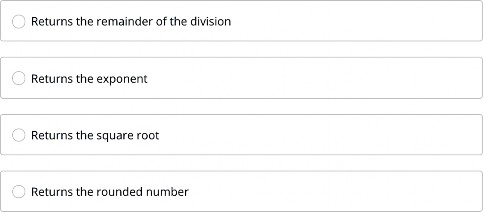2. b = 3

3. # a *= b

Note: The review question comply all the probable questions containing each chapter from the

16

textbook. It gives just the idea about the pattern and layout of the questions and does not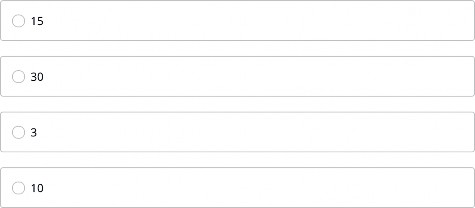2. # b = "World"

3. print(b*3)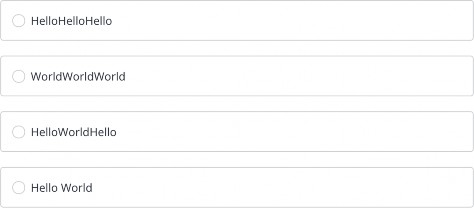3. # c = a + b

4. print(c)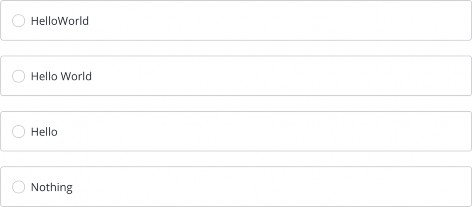3. Given: x = -1

which expression returns True?

A) 0 <= x <= 15

B) x <= -12

C) x < 110

1. All of the above

2. None of the above

4. What is printed by: print(2 and 3)

1. 2

2. -1

3. 3

4. False

5. None of the above

5. Given: x = 0 what is printed by:

if x:

print("Here") else:

print("There")

1. Here

2. There

3. None of the above

6. What is printed by this sequence of instructions?

x = 0

y = 1

z = 1

print(x or (y and not z))

1. 1

2. 0

3. True

4. False

5. None of the above.

7. Given: x = 12

what is printed by: print(0 <= x <= 12)

1. False

2. True

3. 12

4. 0

5. None of the above.

8. Given

b = input("Input a bool (True/False): ")

What is the type of b if True is input at the prompt?

1. float

2. str

3. int

4. bool

5. None of the above.

9. What does this output

>>> 1 + 2 + 3

1. 6

2. 7

3. 5

4. 8

10. Which option is output by this Code?

>>>(4 + 8) / 2

a)8 b)6.0

c)6

d)4

11. Fill in the Blank (Red Color) to make this code correct?

>>> (_5-1)*3

Output: 18

e) +

1. *

2. /

12. Fill in the Blank that will cause a ZeroDivisionError

>>>(17+94)/(-5 + _)

1. -0

2. 0

3. 5

4. -5

13. What is the result of this code?

>> 7%(5//2)

1. 0

2. 7

3. 1

4. None of the Above

14. What is printed by

print(3*2**3)

1. 18

2. 16

3. 24

4. 64

5. None of the above.

15. What is printed by: print(3 and 2)

1. 2

2. 3

3. 2 and 3

4. 5

5. None of the above

16. What is printed by this sequence of instructions?

x = 1

y = 0

z = 1

print(x or (y and not z))

1. 1

2. 0

3. True

4. False

5. None of the above.

MULTIPLE CHOICE ON DATA STRUCTURES

1. # How do you declare a list with the integer elements 1, 2, and 3.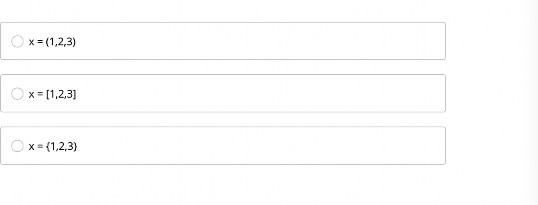2. # What is the main difference between a tuple and a list?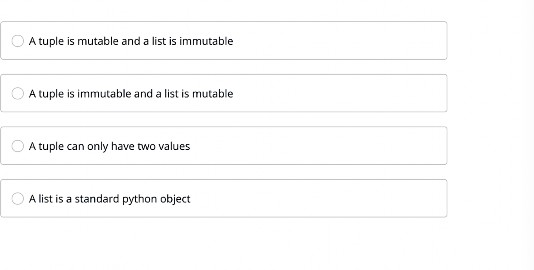3. # What are the pairs in dictionaries called?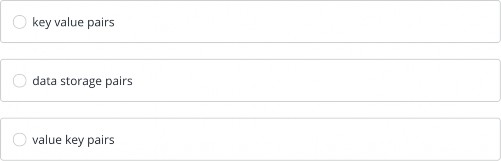4. # How would you access the value stored in key 'x' of a dictionary 'd'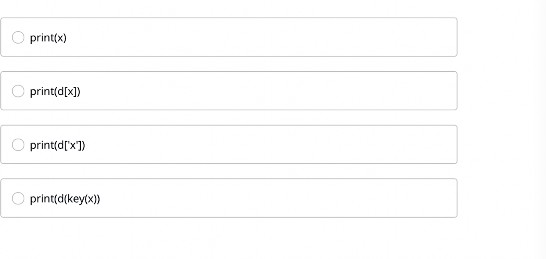# 4. Given: S1 = {1,2,3} and S2 = {2,3,4}

what is the value of S after this operation: S = S1 & S2

A) {1, 2, 3, 4}

B) {2, 3}

C) {1, 4}

1. {}

2. None of the above

5. # What is the correct way to get the number of 'x's in the string 's'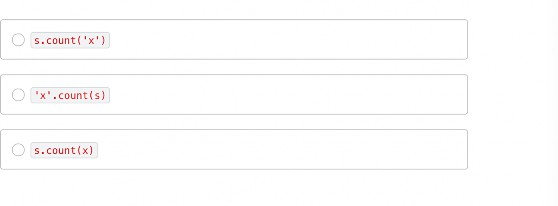6. # What is the correct way get the substring 'abc' from the string s containing 'abcdefg'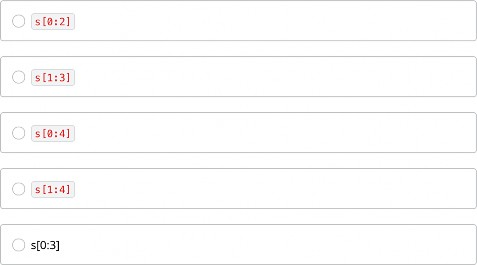7. Given: s = 'spam'

what is printed by: print(s.index('s'))

1. 0

2. ''

3. None

4. -1

5. None of the above

8. Consider this code L1 = ['a','b']

L2 = [1, L1, 2]

L1.append('c') print(L2) # Line 1

What is printed by Line 1?

A) [1, ['a', 'b'], 2]

B) [1, ['a', 'b', 'c'], 2]

C) [1, ['c'], 2]

D) [1, [['a', 'b'],['c']], 2]

E) None of the above

9. What is printed by this sequence of instructions?

L1 = [2,4]

L2 = [3,5]

print(L1 + L2)

A) [2, 4, 3, 5]

B) [2, 3, 4, 5]

C) [[2, 4], [3, 5]]

D) [2, 4, [3, 5]]

E) None of the above.

10. Which of the following prints the first three multiples of three: 3 6 9

1. for i in range(3,12,3): print(i, end=' ')

2. for i in range(1,5): print(3*i, end = ' ')

3. for i in range(3): print(3*i, end=' ')

4. All of the above.

5. None of the above.

11. Given s = "Head, Shoulders, Knees and Toes" which code produces the following?

1. s.split(',') # Note no space

2. s.split(', ') # Note one space after comma

3. s.split().strip()

4. list(s)

5. None of the above.

12. Consider this code L1 = [1,2]

L2 = [3, L1, 4]

L1.append(5) print(L2) # Line 1

What is printed by Line 1?

A) [3, 'L1', 4]

B) [3, [1, 2], 4]

C) [3, , 4]

D) [3, [1, 2, 5], 4]

E) None of the above

13. Given this sequence of instructions:

L1 = [1,2,3]

L2 = ['a',L1[:],'b',L1]

L1.append(4) print(L2)

What is printed?

A) ['a',L1[:],'b',L1]

B) ['a', [1, 2, 3, 4], 'b', [1, 2, 3, 4]]

C) ['a', [1, 2, 3], 'b', [1, 2, 3, 4]]

D) ['a', [1, 2, 3,4], 'b', [1, 2, 3]]

E) None of the above.

14. What is printed by

T1 = (7,8)

T1 += (2,1)

print(T1)

A) ((7, 8), (2, 1))

B) (7, 8, 2, 1)

C) (9, 9)

D) (1, 2)

E) None of the above

15. Given M = {'Bob': 75, 'Alice': 27}

What is printed by print(M['Alice'])

1. 75

2. 27

3. 'Alice'

4. 'Alice': 27

5. None of the above.

16. . The key of a dictionary must be:

1. an int, float, or string

2. an int or string

3. Mutable

4. Immutable

5. None of the above.

## 17. Given: S1 = {1,2,3} and S2 = {1,3,5}

what is the value of S after this operation: S = S1 ^ S2

A) {}

B) {1, 3}

C) {2, 5}

D) {1, 2, 3, 5}

E) None of the above

1. Fill in the missing part of the output

>>>”””First Line Second line”””

First Line   Second line

1. \t

2. \n

3. \r

4. None of the above

2. What line of code produces an error? a) “one” + “2”

b) 3 + 4

c) “7” + ‘eight’

d) ‘5’ + 6

3. What is the output of this code?

>> print(3*’7’)

a) 21

b) 3*’7’

c) 333

d) 777

4. What is printed by this sequence of instructions

L1 = [6, 3, 7, 9, 1]

L2 = sorted(L1) print(L1)

A) [9, 7, 6, 3, 1]

B) [6, 3, 7, 9, 1]

C) [1, 3, 6, 7, 9]

1. None

2. None of the above.

## 22. Given D = {'x': 2, 'y': 4}

What is printed by

print(D['x'])

1. 2

2. 4

C) 'x': 2

1. 'x'

2. None of the above.

1. What is printed by

T1 = (1,2)

T2 = (7,8)

print(T2+T1)

A) ((7, 8), (1, 2))

B) (7, 8, 1, 2)

C) (10, 8)

D) (7, 8) + (1, 2)

E) None of the above

2. Given s = "Twinkle, Twinkle, Little Star" which code produces the following?

['Twinkle', 'Twinkle', 'Little Star']

1. list(s)

2. s.split().strip()

3. s.split(', ') # one space character after comma

4. s.split(',') # no space character

5. None of the above.

3. What is printed by this sequence of instructions?

L1 = [3,5]

L2 = [2,4]

print(L1 + L2)

A) [2, 3, 4, 5]

B) [3, 5, 2, 4]

C) [[3, 5], [2, 4]]

D) [3, 5, [2, 4]]

E) None of the above.

MULTIPLE CHOICE ON CONTROL FLOW

1. # What is the result of this conditional statement?

1. x = 3

2. if x == 4:

2. else:

3. # print("False")

1. True

2. False

3. Error

4. None of the Above

2. B, C, D = 0, 0, 0

for A in [ 0, 1, 3, 5, 7, 9 ]:

if A :

if A%2 == A:

B += 1

else:

C += 1

if A%3 == 0: D += 1

break

print( A ) # Line 1 print( B ) # Line 2 print( C ) # Line 3 print( D ) # Line 4

1. . What is printed by Line 1 of Figure 2?

1. 0

2. 1

3. 2

4. 3

5. None of the above

2. What is printed by Line 2 of Figure 2?

1. 0

2. 1

3. 2

4. 3

5. None of the above

3. What is printed by Line 3 of Figure 2?

1. 0

2. 1

3. 2

4. 3

5. None of the above

4. What is printed by Line 4 of Figure 2?

1. 0

2. 1

3. 2

4. 3

5. None of the above

1. What is printed by? a = 30

if a == 3 or not(a < 20): print("Yes")

else:

print("No")

1. Yes

2. No

3. a is 3

4. None

5. None of the above.

2. What is printed by? a = 20

if a == 3 or not(a < 10): print("No")

else:

print("Yes")

1. Yes

2. No

3. a is 3

4. None

5. None of the above

MULTIPLE CHOICE ON TYPECASTING AND ESCAPE SEQUENCES

1. # What is the newline escape sequence?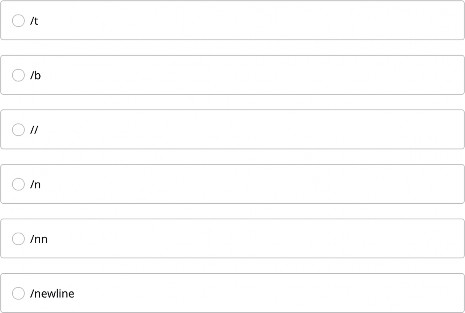2. # What is the correct way to convert an integer to a string and store it in the variable 'result'?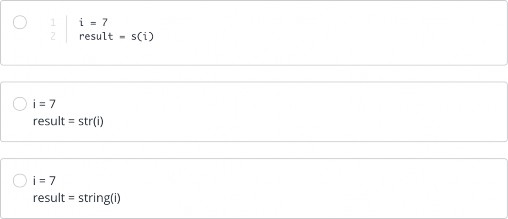# d) “82”

أنت الان في اول موضوع